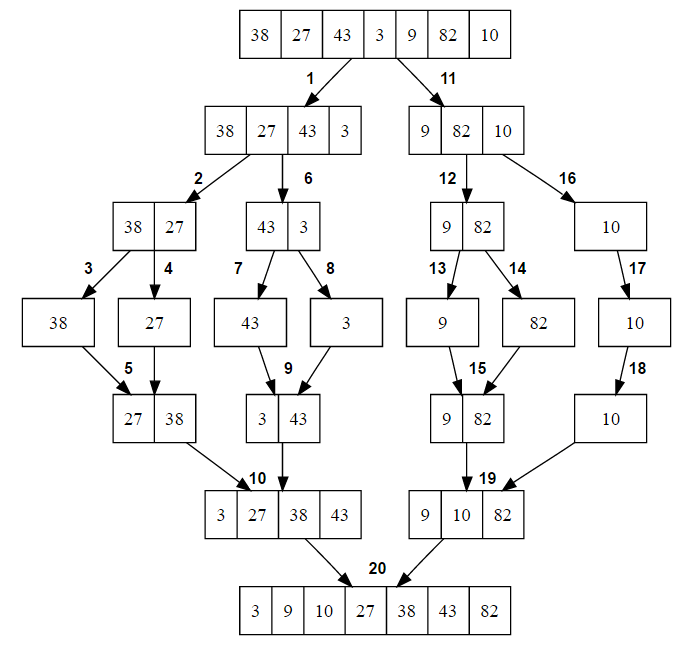# Merge Sort Algorithm

Given an array of integers, sort it using merge sort algorithm.

Merge sort is an efficient in-place sorting algorithm which produces a stable sort, which means that if two elements have the same value, they holds same relative position in the output as they did in the input. In other words, the relative order of elements with equal values is preserved in the sorted output. Merge sort is a comparison sort which means that it can sort any input for which a less-than relation is defined.

### How Merge sort works?

Merge sort is a divide and conquer algorithm. Like all divide and conquer algorithms, merge sort divides a large array into two smaller subarrays and then recursively sort the subarrays. Basically, there are two steps are involved in whole process –

1. Divide the unsorted array into n subarrays, each of size 1 (an array of size 1 is considered sorted).

2. Repeatedly merge subarrays to produce new sorted subarrays until only 1 subarray is left which would be our sorted array.

Below diagram shows top-down view of recursive merge sort algorithm used to sort an array of 7 integers.Below is C and Java implementation of the Merge Sort Algorithm:

## C

Output:

-50 -41 -34 -23 -21 -11 5 9 10 19 26 33 35 40 49

## Java

Output:

-2 0 3 5 12 18 24

## Merge Sort Performance:

Worst case time complexity of merge sort is O(nlog(n)). The recurrence relation is

T(n) = 2T(n/2) + cn = O(nlog(n))

The recurrence basically summaries merge sort algorithm – Sort two lists of half the size of the original list, and add the n steps taken to merge the resulting two lists.

Auxiliary space required by it is O(n).

Also See:

References: https://en.wikipedia.org/wiki/Merge_sort(3 votes, average: 5.00 out of 5)Loading...

Please use our online compiler to post code in comments. To contribute, get in touch with us.
Like us? Please spread the word and help us grow. Happy coding 🙂